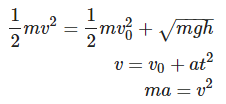# Dimensional analysis worksheet

In this page we have Dimensional analysis worksheet . Hope you like them and do not forget to like , social share and comment at the end of the page.

Question 1 Show that expression $v={{v}_{0}}+ at$ is dimensionally correct, where $v$ and ${{v}_{0}}$ represent velocities and $a$ is acceleration and $t$ represents time.

Here in this question , we need to compare the dimension of the Left hand side and right hand side
LHS
$v= [LT^{-1}]$
RHS
${{v}_{0}}+ at = [LT^{-1}] + [LT^{-2}][T]=[LT^{-1}] + [LT^{-1}] =[LT^{-1}]$
So, the dimensions on both the sides are same
So , we can say that expression $v={{v}_{0}}+ at$ is dimensionally correct

Question 2 Each of the following equations was given by a student during an examination.Do the dimensional analysis of each equation and explain why the equations cannot be correct.

a. The expression is $\frac {1}{2}mv^2 = \frac {1}{2}mv_0^2 + \sqrt {mgh}$
LHS
$\frac {1}{2}mv^2 = M(LT^{-1})^2= ML^2T^{-2}$
RHS
$\frac {1}{2}mv_0^2 + \sqrt {mgh}=M(LT^{-1})^2 + [ML^2T^{-2}]^{1/2} = ML^2T^{-2} + M^{1/2}LT^{-1}$
Now this cannnot be correct as we cannot add quantities of two different dimension.
So this equation is incorrect

b.The expression is $v= v_0 + at^2$
LHS
$v= [LT^{-1}]$
RHS
$v_0 + at^2 = [LT^{-1}] + [LT^{-2}]T^2= [LT^{-1}] + [L]$
Now this cannnot be correct as we cannot add quantities of two different dimension.
So this equation is incorrect

c.The expression is $ma= v^2$
LHS
$ma= [M][LT^{-2}]=[MLT^{-2}]$
RHS
$v^2 = [L^2T^{-2}]$
Now dimension of LHS is not equal to dimension of RHS
So this equation is incorrect

Question 3 Suppose that the displacement of an object is related to time according to the expression $x=B{{t}^{2}}$ . What are the dimensions of $B$?

Here $x=B{{t}^{2}}$
or
$B= \frac {x}{t^2}$
Therefore dimension of B will be
=$\frac {[L]}{[T^{2}]}= [LT^{-2}]$

Question 4 Find the SI unit of pressure.

SI unit of pressure is pascal

Question 5 (a) Is it possible for two quantities to have the same dimensions but different units.
(b) Is it possible for two quantities to have same units but different dimensions?

a. This is possible . Example Work and Torque have different unit Joule and Newton-meter but the dimension $[ML^2T^{-1}]$ is same
b. No it is not possible.

Question 6 Suppose we are told that the acceleration ‘a’ of a particle moving with uniform speed ‘v’ in a circle of radius ‘r’ is proportional to some power of r, say rn, and some power of vm. How can we determine the value of n and m.

We can write
$a \; \alpha \; v^m r^n$
$a= Kv^m r^n$
Where K is the dimensionless constant
Now writing dimension of various quantities in the equation
$[LT^{-2}]=[LT^{-1}]^m [L]^n$
$[LT^{-2}]=[L^{m+n} T^{-m}]$
Comparing the dimensions of similar quantities on both the sides, we get
$m+n=1$
$-m=-2$ or $m=2$
Therefore 2+n=1 or n=-1
Hence the values of m and n are 2 and -1 respectively

Question 7 The mass of a solid cube is 856 g, and each edge has a length of 5.35 cm. Determine the density $\rho$ of the cube in basic SI units.

Now $\rho = \frac {M}{V}$
Here M= 856 g = .856 kg
$V= (5.35)^3 cm^3= (5.35)^3 (1/100)^3 m^3$
$\rho = \frac {.856}{ (5.35)^3 (1/100)^3} kg/m^3 = 5590 kg/m^3$

Question 8 The period ‘T’ of a simple pendulum is measured in time units and is described by
$T=2\pi \sqrt{\frac{l}{g}}$ Where l is the length of the pendulum and ‘g’ is the free fall acceleration in units of length divided by square of time. Show that this equation is dimensionally correct.

$T=2\pi \sqrt{\frac{l}{g}}$
LHS
$T = [T]$
RHS
$2\pi \sqrt{\frac{l}{g}} = (\frac {[L]}{[LT^{-2}]})^{1/2} = ([T^{2}])^{1/2} =[T]$
So, this equation is dimensionally consistent

Question 9 Estimate the average mass density of a sodium atom assuming its size to be about 2.5 Å. (Use the known values of Avogadro’s number and the atomic mass of sodium). Compare it with the density of sodium in its crystalline phase: 970 kg m-3. Are the two densities of the same order of magnitude? If so, why?
Question 10 In the following x is in meters, t in seconds, v in m/s and a is in m/s2. Find the SI unit of each combination.
a. $\frac{{{v}^{2}}}{xa}$
b.$\sqrt{\frac{x}{a}}$
c. $\frac{1}{2}at^2$

a. $\frac{{{v}^{2}}}{xa}$
$= \frac {(m/s)^2}{m m/s^2}= \frac {(m/s)^2}{(m/s)^2}$ =dimensionless

b.
$\sqrt {\frac {m}{m/s^2}}= \sqrt {s^2} =s$

c.Here constant 1/2 does not have any units
$(m/s^2) s^2 = m$